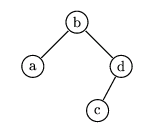시간 제한메모리 제한제출정답맞힌 사람정답 비율
1 초 128 MB54480.000%

## 문제

A binary tree can either be empty or consist of one vertex, with two trees linked to it. These two trees are called a left and a right subtree. In each vertex there is one letter from the English alphabet. The vertex which is not a subtree of any vertex, is called a root. We say that a tree is a Binary Search Tree (BST) if for each vertex the following condition is satisfied: all letters in the left subtree precede in alphabetical order the letter in the root, and all letters from the right subtree follow the letter in the root. A code of a BST is:

either an empty string (containing 0 letters) when the tree is empty
or a string beginning with the letter from the root, followed by the code of the left subtree, followed by the code of the right subtree.

Let us consider all -vertex BSTs containing in their vertices k initial letters of the English alphabet. Suppose we have a list of these codes written in alphabetical order. (n,k)-code is the n-th code on this list.

For example :

There are exactly fourteen 4-vertex BSTs. These are (in alphabetical order):

The string badc is the (7,4)-code and it corresponds to the BST printed below:Write a program which:

• reads the numbers  and  from the standard input,
• finds the (n,k)-code,
• writes it to the standard output.

## 입력

In the first and only line of the standard input there are exactly two positive integers n and k, separated by a single space, 1 ≤ k ≤ 19. The number n is not greater than the number of codes of BST with k vertices.

## 출력

In the first and only line of the standard output there should be exactly one word (written in small letters) being the (n,k)-code.

## 예제 입력 1

11 4


## 예제 출력 1

dacb